## Greatest Common Divisor

The greatest common divisor ofandGCD(), sometimes written, is the largest Divisor common toand. Symbolically, let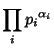(1)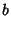(2)

Then the greatest common divisor is given by(3)

where min denotes the Minimum. The GCD is Distributive(4)(5)

and Associative(6)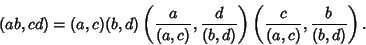(7)

Ifand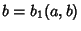, then(8)

soandandare said to be Relatively Prime. The GCD is also Idempotent(9)

Commutative(10)

and satisfies the Absorption Law(11)

The probability that two Integers picked at random are Relatively Prime is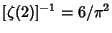, whereis the Riemann Zeta Function. Polezzi (1997) observed that, whereis the number of Lattice Points in the Plane on the straight Line connecting the Vectors (0, 0) and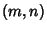(excludingitself). This observation is intimately connected with the probability of obtaining Relatively Prime integers, and also with the geometric interpretation of a Reduced Fraction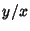as a string through a Lattice of points with ends at (1,0) and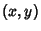. The pegs it presses againstgive alternate Convergents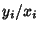of the Continued Fraction for, while the other Convergents are obtained from the pegs it presses against with the initial end at (0, 1).

Knuth showed that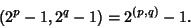(12)

The extended greatest common divisor of two Integersandcan be defined as the greatest common divisorofandwhich also satisfies the constraint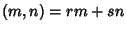forand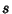given Integers. It is used in solving Linear Diophantine Equations.

Polezzi, M. A Geometrical Method for Finding an Explicit Formula for the Greatest Common Divisor.'' Amer. Math. Monthly 104, 445-446, 1997.